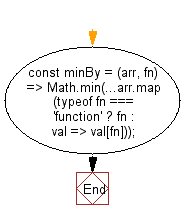# JavaScript: Get the minimum value of an array, after mapping each element to a value using the provided function

## JavaScript fundamental (ES6 Syntax): Exercise-177 with Solution

Write a JavaScript program to get the minimum value of an array, after mapping each element to a value using the provided function.

• Use Array.prototype.map() to map each element to the value returned by fn.
• Use Math.min() to get the minimum value.

Sample Solution:

JavaScript Code:

``````//#Source https://bit.ly/2neWfJ2
const minBy = (arr, fn) => Math.min(...arr.map(typeof fn === 'function' ? fn : val => val[fn]));
console.log(minBy([{ n: 4 }, { n: 2 }, { n: 8 }, { n: 6 }], o => o.n));
console.log(minBy([{ n: 4 }, { n: 2 }, { n: 8 }, { n: 6 }], 'n'));
```
```

Sample Output:

```2
2
```

Pictorial Presentation:Flowchart:Live Demo:

See the Pen javascript-basic-exercise-177-1 by w3resource (@w3resource) on CodePen.

Improve this sample solution and post your code through Disqus

What is the difficulty level of this exercise?

Test your Programming skills with w3resource's quiz.

﻿